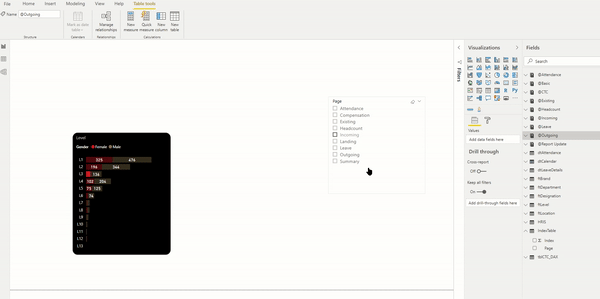top of page
Search

# Custom Sorting in Power BIWhile using dimensions in any visuals, you can sort it either by dimension or the measures, and calculations used in it.In the case of dimensions, it sorts in alphabetical order.

There are scenarios where we want to sort the dimension in a predefined order. How we can customize the sorting in Power BI visuals?

Let us take an example of the following table:The index column represents the order of the sorting.

When used in a visual, it sorts alphabetically.To change it according to the Index column, apply the following steps:

Select the column “Page” > Column Tools > Sort by Column > Select “Index”bottom of page